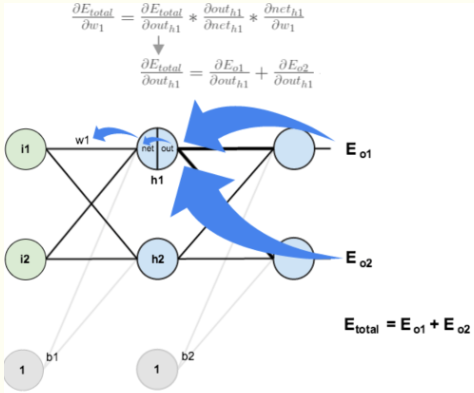# 一、望尽天涯路

1974年，Paul Werbos首次给出了如何训练一般网络的学习算法—$back propagation$。这个算法可以高效的计算每一次迭代过程中的梯度，让以上我们的推导得以实现！然而不巧的是，在当时整个人工神经网络社群中无人知晓Paul所提出的学习算法。直到80年代中期，$BP$算法才重新被David Rumelhart、Geoffrey Hinton及Ronald Williams、David Parker和Yann LeCun独立发现，并获得了广泛的注意，引起了人工神经网络领域研究的第二次热潮，反向传播正式出现在大众面前。

• 第一个问题 为什么要理解“反向传播”？

• 第二个问题 反向传播算法为什么要“反向”？$\frac{\partial y}{\partial x} \approx \frac{f(x+ \Delta x)-f(x)}{\Delta x}$

# 二、一千个人眼中有一千个哈姆雷特

#### 1.【知乎高赞回答：如何直观地解释 backpropagation 算法？—— Anonymous】

$BackPropagation$(反向传播)算法是多层神经网络的训练中举足轻重的算法。简单的理解，它的确就是复合函数的链式法则，但其在实际运算中的意义比链式法则($chain rule$)要大的多。$\frac{\partial e}{\partial a}=\frac{\partial e}{\partial c} \cdot \frac{\partial c}{\partial a}$
$\frac{\partial e}{\partial b}=\frac{\partial e}{\partial c} \cdot \frac{\partial c}{\partial b}+\frac{\partial e}{\partial d} \cdot \frac{\partial d}{\partial b}$#### 2.【博客园博客：一文弄懂神经网络中的反向传播法—BackPropagation——Charlotte77】$E_{\text {total}}=\sum \frac{1}{2}(\text {target}-\text { output })^{2}$

$E_{t o t a l}=E_{o 1}+E_{o 2}$
$w5$$w1$为例：$w_{5}^{+}=w_{5}-\eta * \frac{\partial E_{t o t a l}}{\partial w_{5}}$$w_{1}^{+}=w_{1}-\eta * \frac{\partial E_{t o t a l}}{\partial w_{1}}$

import random
import math

#   参数解释：
#   "pd_" ：偏导的前缀
#   "d_" ：导数的前缀
#   "w_ho" ：隐含层到输出层的权重系数索引
#   "w_ih" ：输入层到隐含层的权重系数的索引

class NeuralNetwork:
LEARNING_RATE = 0.5

def __init__(self, num_inputs, num_hidden, num_outputs, hidden_layer_weights = None, hidden_layer_bias = None, output_layer_weights = None, output_layer_bias = None):
self.num_inputs = num_inputs

self.hidden_layer = NeuronLayer(num_hidden, hidden_layer_bias)
self.output_layer = NeuronLayer(num_outputs, output_layer_bias)

self.init_weights_from_inputs_to_hidden_layer_neurons(hidden_layer_weights)
self.init_weights_from_hidden_layer_neurons_to_output_layer_neurons(output_layer_weights)

def init_weights_from_inputs_to_hidden_layer_neurons(self, hidden_layer_weights):
weight_num = 0
for h in range(len(self.hidden_layer.neurons)):
for i in range(self.num_inputs):
if not hidden_layer_weights:
self.hidden_layer.neurons[h].weights.append(random.random())
else:
self.hidden_layer.neurons[h].weights.append(hidden_layer_weights[weight_num])
weight_num += 1

def init_weights_from_hidden_layer_neurons_to_output_layer_neurons(self, output_layer_weights):
weight_num = 0
for o in range(len(self.output_layer.neurons)):
for h in range(len(self.hidden_layer.neurons)):
if not output_layer_weights:
self.output_layer.neurons[o].weights.append(random.random())
else:
self.output_layer.neurons[o].weights.append(output_layer_weights[weight_num])
weight_num += 1

def inspect(self):
print('------')
print('* Inputs: {}'.format(self.num_inputs))
print('------')
print('Hidden Layer')
self.hidden_layer.inspect()
print('------')
print('* Output Layer')
self.output_layer.inspect()
print('------')

def feed_forward(self, inputs):
hidden_layer_outputs = self.hidden_layer.feed_forward(inputs)
return self.output_layer.feed_forward(hidden_layer_outputs)

def train(self, training_inputs, training_outputs):
self.feed_forward(training_inputs)

# 1. 输出神经元的值
pd_errors_wrt_output_neuron_total_net_input =  * len(self.output_layer.neurons)
for o in range(len(self.output_layer.neurons)):

# ∂E/∂zⱼ
pd_errors_wrt_output_neuron_total_net_input[o] = self.output_layer.neurons[o].calculate_pd_error_wrt_total_net_input(training_outputs[o])

# 2. 隐含层神经元的值
pd_errors_wrt_hidden_neuron_total_net_input =  * len(self.hidden_layer.neurons)
for h in range(len(self.hidden_layer.neurons)):

# dE/dyⱼ = Σ ∂E/∂zⱼ * ∂z/∂yⱼ = Σ ∂E/∂zⱼ * wᵢⱼ
d_error_wrt_hidden_neuron_output = 0
for o in range(len(self.output_layer.neurons)):
d_error_wrt_hidden_neuron_output += pd_errors_wrt_output_neuron_total_net_input[o] * self.output_layer.neurons[o].weights[h]

# ∂E/∂zⱼ = dE/dyⱼ * ∂zⱼ/∂
pd_errors_wrt_hidden_neuron_total_net_input[h] = d_error_wrt_hidden_neuron_output * self.hidden_layer.neurons[h].calculate_pd_total_net_input_wrt_input()

# 3. 更新输出层权重系数
for o in range(len(self.output_layer.neurons)):
for w_ho in range(len(self.output_layer.neurons[o].weights)):

# ∂Eⱼ/∂wᵢⱼ = ∂E/∂zⱼ * ∂zⱼ/∂wᵢⱼ
pd_error_wrt_weight = pd_errors_wrt_output_neuron_total_net_input[o] * self.output_layer.neurons[o].calculate_pd_total_net_input_wrt_weight(w_ho)

# Δw = α * ∂Eⱼ/∂wᵢ
self.output_layer.neurons[o].weights[w_ho] -= self.LEARNING_RATE * pd_error_wrt_weight

# 4. 更新隐含层的权重系数
for h in range(len(self.hidden_layer.neurons)):
for w_ih in range(len(self.hidden_layer.neurons[h].weights)):

# ∂Eⱼ/∂wᵢ = ∂E/∂zⱼ * ∂zⱼ/∂wᵢ
pd_error_wrt_weight = pd_errors_wrt_hidden_neuron_total_net_input[h] * self.hidden_layer.neurons[h].calculate_pd_total_net_input_wrt_weight(w_ih)

# Δw = α * ∂Eⱼ/∂wᵢ
self.hidden_layer.neurons[h].weights[w_ih] -= self.LEARNING_RATE * pd_error_wrt_weight

def calculate_total_error(self, training_sets):
total_error = 0
for t in range(len(training_sets)):
training_inputs, training_outputs = training_sets[t]
self.feed_forward(training_inputs)
for o in range(len(training_outputs)):
total_error += self.output_layer.neurons[o].calculate_error(training_outputs[o])

class NeuronLayer:
def __init__(self, num_neurons, bias):

# 同一层的神经元共享一个截距项b
self.bias = bias if bias else random.random()

self.neurons = []
for i in range(num_neurons):
self.neurons.append(Neuron(self.bias))

def inspect(self):
print('Neurons:', len(self.neurons))
for n in range(len(self.neurons)):
print(' Neuron', n)
for w in range(len(self.neurons[n].weights)):
print('  Weight:', self.neurons[n].weights[w])
print('  Bias:', self.bias)

def feed_forward(self, inputs):
outputs = []
for neuron in self.neurons:
outputs.append(neuron.calculate_output(inputs))
return outputs

def get_outputs(self):
outputs = []
for neuron in self.neurons:
outputs.append(neuron.output)
return outputs

class Neuron:
def __init__(self, bias):
self.bias = bias
self.weights = []

def calculate_output(self, inputs):
self.inputs = inputs
self.output = self.squash(self.calculate_total_net_input())
return self.output

def calculate_total_net_input(self):
total = 0
for i in range(len(self.inputs)):
total += self.inputs[i] * self.weights[i]

# 激活函数sigmoid
def squash(self, total_net_input):
return 1 / (1 + math.exp(-total_net_input))

def calculate_pd_error_wrt_total_net_input(self, target_output):
return self.calculate_pd_error_wrt_output(target_output) * self.calculate_pd_total_net_input_wrt_input();

# 每一个神经元的误差是由平方差公式计算的
def calculate_error(self, target_output):
return 0.5 * (target_output - self.output) ** 2

def calculate_pd_error_wrt_output(self, target_output):
return -(target_output - self.output)

def calculate_pd_total_net_input_wrt_input(self):
return self.output * (1 - self.output)

def calculate_pd_total_net_input_wrt_weight(self, index):
return self.inputs[index]

# 文中的例子:

nn = NeuralNetwork(2, 2, 2, hidden_layer_weights=[0.15, 0.2, 0.25, 0.3], hidden_layer_bias=0.35, output_layer_weights=[0.4, 0.45, 0.5, 0.55], output_layer_bias=0.6)
for i in range(10000):
nn.train([0.05, 0.1], [0.01, 0.09])
print(i, round(nn.calculate_total_error([[[0.05, 0.1], [0.01, 0.09]]]), 9))

#另外一个例子，可以把上面的例子注释掉再运行一下:

# training_sets = [
#     [[0, 0], ],
#     [[0, 1], ],
#     [[1, 0], ],
#     [[1, 1], ]
# ]

# nn = NeuralNetwork(len(training_sets), 5, len(training_sets))
# for i in range(10000):
#     training_inputs, training_outputs = random.choice(training_sets)
#     nn.train(training_inputs, training_outputs)
#     print(i, nn.calculate_total_error(training_sets))


#### 3.Principles of training multi-layer neural network using backpropagation# 三、天下谁人不识君

1)链式法则的直观理解之所以可以链式法则，是因为梯度直观上理解就是一阶近似，所以梯度可以理解成某个变量或某个中间变量对输出影响的敏感度的系数，这种理解在一维情况下的直观帮助可能并不是很大，但是到了高维情况，当链式法则从乘法变成了$Jacobian$矩阵乘法的时候，这个理解起来就形象多了。神经网络中的链式法则恰好都几乎是高维的。

2)$Computational graph$。其实就是计算代数中的一个最基础办法，从计算机的角度来看还有点动态规划的意思。其优点是表达式给定的情况下对复合函数中所有变量进行快速求导，这正好是神经网络尤其是深度学习的场景。